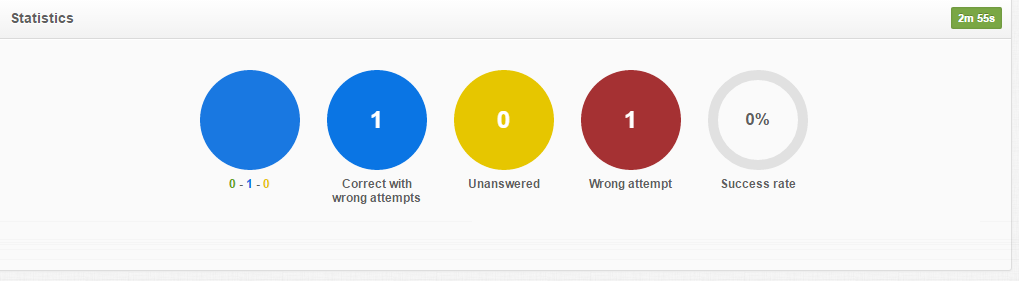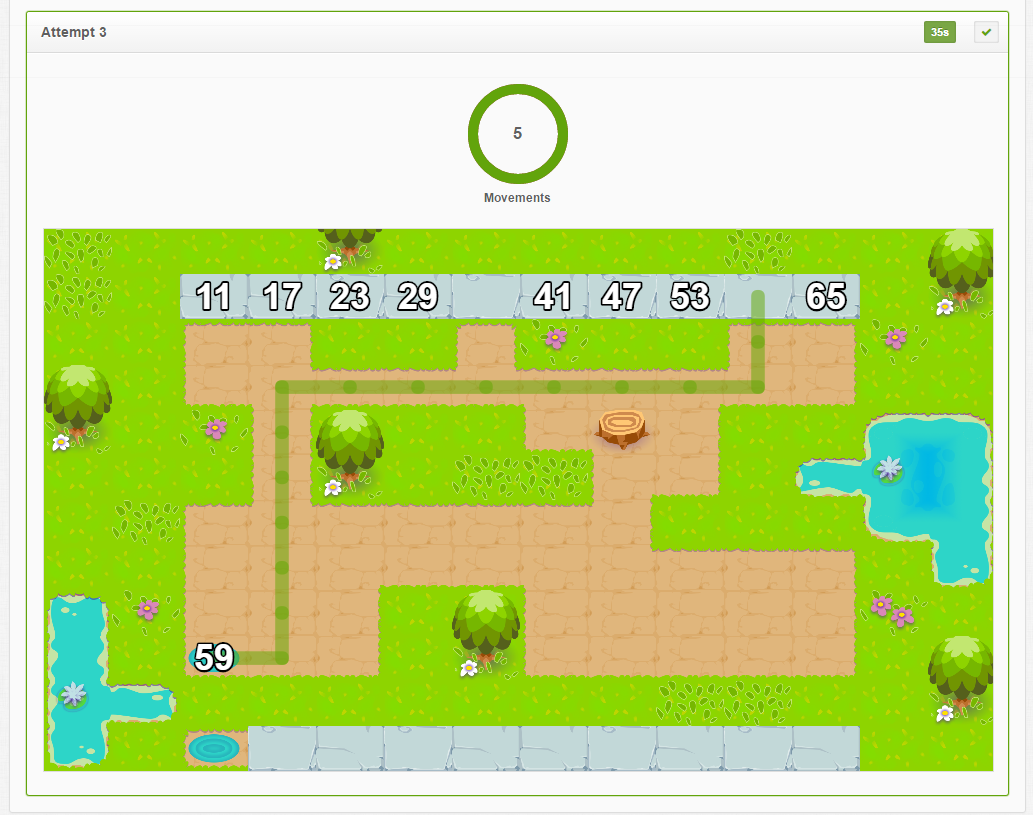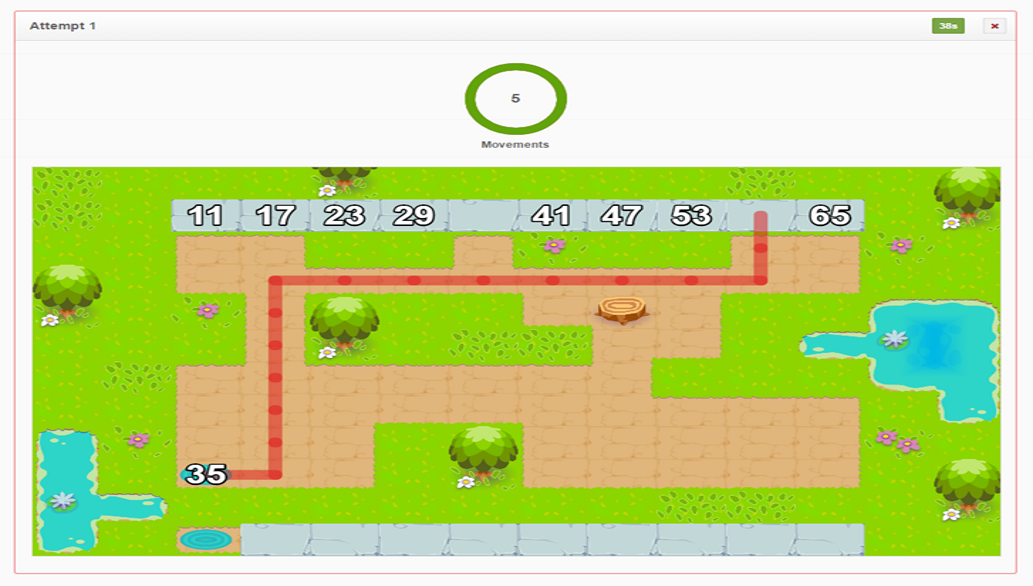### Doffies

“Doffies” offers an engaging ordering and number sequences game that also promotes motor planning and execution skills. The student must recognize a number sequence and drive a “Doffy” – a purple funny avatar creature created by dough. The Doffy holds a number and walks through a maze in order to line it up next to other Doffies to correctly the sequence of numbers up to 100. In order to drive a Doffy, the student has to make jumping, squat and side walking movements which improve visual motor coordination skills and balance. This game has different levels which can be matched to a child's mathematical ability level and motor skills.

The teacher/therapist can adjust the game by choosing the learning content including the number sequences that can appear in forward and backward order. This reinforces the child’s knowledge of multiples (2, 3, 4, 5, and 10) and number order. The teacher can also modify the complexity level of the maze thus promoting the visual perception and motor coordination skills of the student.

## Supported goals in Common CoreCaliforniaFloridaNevadaNew YorkNorth CarolinaPennsylvaniaSouth CarolinaTenesseeTexasVirginia

CCSS.MATH.CONTENT.K.CC.A.2
Math
Count forward beginning from a given number within the known sequence (instead of having to begin at 1).
CCSS.MATH.CONTENT.4.NF.C.6
Math
Use decimal notation for fractions with denominators 10 or 100. For example, rewrite 0.62 as 62/100; describe a length as 0.62 meters; locate 0.62 on a number line diagram.
CA.MATH.K.CC.2
Math
Know number names and the count sequence. Count forward beginning from a given number within the known sequence (instead of having to begin at 1).
CA.MATH.4.NF.6
Math
Understand decimal notation for fractions, and compare decimal fractions. Use decimal notation for fractions with denominators 10 or 100. For example, rewrite 0.62 as 62/100 ; describe a length as 0.62 meters; locate 0.62 on a number line diagram. (Grade 4 expectations in this domain are limited to fractions with denominators 2, 3, 4, 5, 6, 8, 10, 12, and 100.)
MAFS.K.CC.1.AP.2a
Math
Rote count forward from a given number (instead of having to begin at 1)
MAFS.4.NF.3.6
Math
Use decimal notation for fractions with denominators 10 or 100. For example, rewrite 0.62 as 62/100; describe a length as 0.62 meters; locate 0.62 on a number line diagram.
NVAC.MATH.K.CC.A.2
Math
Know number names and the count sequence. Count forward beginning from a given number within the known sequence (instead of having to begin at 1).
NVAC.MATH.4.NF.C.6
Math
Understand decimal notation for fractions, and compare decimal fractions. Use decimal notation for fractions with denominators 10 or 100. For example, rewrite 0.62 as 62/100 ; describe a length as 0.62 meters; locate 0.62 on a number line diagram. (Grade 4 expectations in this domain are limited to fractions with denominators 2, 3, 4, 5, 6, 8, 10, 12, and 100.)
NY.MATH.4.NF.6
Math
Use decimal notation for fractions with denominators 10 or 100. For example, rewrite 0.62 as 62/100; describe a length as 0.62 meters; locate 0.62 on a number line diagram.
NY.MATH.K.CC.2
Math
Count forward beginning from a given number within the known sequence (instead of having to begin at 1).
NC.MATH.K.CC.2
Math
Count forward beginning from a given number within the known sequence, instead of having to begin at 1.
NC.MATH.4.NF.6
Math
Use decimal notation to represent fractions. Express, model and explain the equivalence between fractions with denominators of 10 and 100. Use equivalent fractions to add two fractions with denominators of 10 or 100. Represent tenths and hundredths with models, making connections between fractions and decimals.
PA.CC.MATH.4.NF.C.6
Math
Use decimal notation for fractions with denominators 10 or 100. For example, rewrite 0.62 as 62/100; describe a length as 0.62 meters; locate 0.62 on a number line diagram.
PA.CC.MATH.K.CC.A.2
Math
Count forward beginning from a given number within the known sequence (instead of having to begin at 1).
SC.MATH.4.NSF.6
Math
Write a fraction with a denominator of 10 or 100 using decimal notation, and read and write a decimal number as a fraction
SC.MATH.K.NS.2
Math
Count forward by ones beginning from any number less than 100.
No supported goals.
TEKS.MATH.4.2.G
Math
Relate decimals to fractions that name tenths and hundredths.
VASOL.MATH.4.NNS.3d
Math
The student will given a model, write the decimal and fraction equivalents
VASOL.MATH.K.NNS.4b
Math
The student will identify one more than a number and one less than a number.

## Settings

### I CAN statement

Music

Disable/enable background music

• On
• Off
Sound effects

Disable/enable sound effects while selecting an item.

• On
• Off

I CAN understand that there are sounds when I choose an item.

Select hand
• Left
• Right

I CAN select the “Play Button” with my left hand.

Time to select item

Time to remain stable to select an item

Reduce the time if the child shows stability

• 0.5 sec
• 1 sec
• 1.5 sec
• 2 secs
• 2.5 secs
• 3 secs

I CAN select the “Play Button”, keeping my hand stable for 3 seconds.

Timer

Time to complete the number line.

Disable this if the student feels pressure

• On
• Off

I CAN answer 1 question in 3 min.

Timer value
• 2 mins
• 2.5 mins
• 3 mins
• 3.5 mins
• 4 mins
• 4.5 mins
• 5 mins
• 5.5 mins
• 6 mins
• 6.5 mins
• 7 mins
• 7.5 mins
• 8 mins
Number of questions

This shows the number of questions/stages for each game.

• 1 question
• 2 questions
• 3 questions
• 4 questions
• 5 questions

I CAN answer 5 questions/stages correctly.

Path difficulty

This option sets the complexity of the maze and adds extra difficulty and obstacles in finding the correct path.

• Easy
• Hard

I CAN win a game in a hard level.

I CAN plan the route I have to follow within the maze.

Missing sequence numbers

This determines the number of possible missing numbers that the student can complete in a number line.

• 2 numbers
• 3 numbers
• 4 numbers

I CAN find and complete 4 missing numbers in a number line.

Number type

Teachers can determine if the number line will include natural, decimal or no numbers.

• Natural
• Decimal
• None

I CAN lead decimal numbers in the right position of a number line.

Content difficulty

This option makes it harder to select the right answer reinforcing the knowledge of multiples in a number line.

• Easy
• Medium
• Hard

I CAN win a game in a hard level.

I CAN find the right position of each number in a number line with multiples of 4.

Numeric range

Adjust the range to match the student’s abilities

• 0 - 10
• 0 - 20
• 0 - 50
• 0 - 100

I CAN lead numbers in the right position of a number line that consists of two digit-numbers.

Reverse number sequence

This option enables reverse number sequence, adding extra difficulty in completing the number line.

• On
• Off

I CAN complete a reverse number line.

Game lives

Enable or disable game lives

Game lives are the 3 hearts in the right of the screen.

• On
• Off

I CAN notice that the hearts disappear when I am wrong.

I CAN play the game with game lives.

## ReportsPie chart data

·        This shows statistics of how many times the student complete or not the number sequence and how many times the student gave wrong answer.

·        The Time is shown on the top right corner. The teacher can use the time to tell whether the task is challenging enough for the students.Graph data

·      This shows the number of movements the student made for each completion number. In this case, the student made 5 correct movements (one step left to enter the stage, one step right, one jumping, a second step right, a second jumping).

·      If the completion of the number is correct, the path line is green. Otherwise, if the completion of the number is wrong, the path line is red.

The data can help the teacher to see whether there is a certain path line that the student faces difficulty in finding the route. Teachers can compare the motor planning and the organization in order to find the right path to monitor the progress of the students.